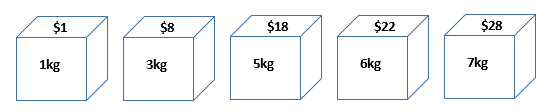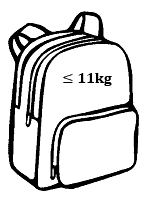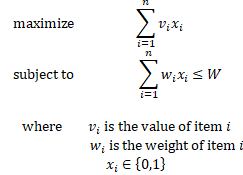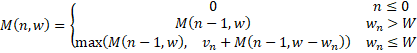### I just announced the new Learn Spring course, focused on the fundamentals of Spring 5 and Spring Boot 2:

>> CHECK OUT THE COURSE

## 1. Introduction

The knapsack problem is a combinatorial optimization problem that has many applications. In this tutorial, we'll solve this problem in Java.

## 2. The Knapsack Problem

In the knapsack problem, we have a set of items. Each item has a weight and a worth value:We want to put these items into a knapsack. However, it has a weight limit:Therefore, we need to choose the items whose total weight does not exceed the weight limit, and their total value is as high as possible. For example, the best solution for the above example is to choose the 5kg item and 6kg item, which gives a maximum value of \$40 within the weight limit.

The knapsack problem has several variations. In this tutorial, we will focus on the 0-1 knapsack problem. In the 0-1 knapsack problem, each item must either be chosen or left behind. We cannot take a partial amount of an item. Also, we cannot take an item multiple times.

## 3. Mathematical Definition

Let's now formalize the 0-1 knapsack problem in mathematical notation. Given a set of n items and the weight limit W, we can define the optimization problem as:This problem is NP-hard. Therefore, there is no polynomial-time algorithm to solve it currently. However, there is a pseudo-polynomial time algorithm using dynamic programming for this problem.

## 4. Recursive Solution

We can use a recursion formula to solve this problem:In this formula, M(n,w) is the optimal solution for n items with a weight limit w. It is the maximum of the following two values:

• The optimal solution from (n-1) items with the weight limit w (excluding the n-th item)
• Value of the n-th item plus the optimal solution from (n-1) items and w minus weight of the n-th item (including the n-th item)

If the weight of the n-th item is more than the current weight limit, we don't include it. Therefore, it is in the first category of the above two cases.

We can implement this recursion formula in Java:

``````int knapsackRec(int[] w, int[] v, int n, int W) {
if (n <= 0) {
return 0;
} else if (w[n - 1] > W) {
return knapsackRec(w, v, n - 1, W);
} else {
return Math.max(knapsackRec(w, v, n - 1, W), v[n - 1]
+ knapsackRec(w, v, n - 1, W - w[n - 1]));
}
}
``````

In each recursion step, we need to evaluate two sub-optimal solutions. Therefore, the running time of this recursive solution is O(2n).

## 5. Dynamic Programming Solution

Dynamic programming is a strategy for linearizing otherwise exponentially-difficult programming problems. The idea is to store the results of subproblems so that we do not have to re-compute them later.

We can also solve the 0-1 knapsack problem with dynamic programming. To use dynamic programming, we first create a 2-dimensional table with dimensions from 0 to n and 0 to W. Then, we use a bottom-up approach to calculate the optimal solution with this table:

``````int knapsackDP(int[] w, int[] v, int n, int W) {
if (n <= 0 || W <= 0) {
return 0;
}

int[][] m = new int[n + 1][W + 1];
for (int j = 0; j <= W; j++) {
m[j] = 0;
}

for (int i = 1; i <= n; i++) {
for (int j = 1; j <= W; j++) {
if (w[i - 1] > j) {
m[i][j] = m[i - 1][j];
} else {
m[i][j] = Math.max(
m[i - 1][j],
m[i - 1][j - w[i - 1]] + v[i - 1]);
}
}
}
return m[n][W];
}
``````

In this solution, we have a nested loop over the item number n and the weight limit W. Therefore, it's running time is O(nW).

## 6. Conclusion

In this tutorial, we showed a math definition of the 0-1 knapsack problem. Then we provided a recursive solution to this problem with Java implementation. Finally, we used dynamic programming to solve this problem.

As always, the source code for the article is available over on GitHub.

### I just announced the new Learn Spring course, focused on the fundamentals of Spring 5 and Spring Boot 2:

>> CHECK OUT THE COURSE
Inline FeedbacksGeoffrey De Smet
1 year ago

Neither the recursive solution nor the dynamic programming solution will scale to 1000 items, because Knapsack is NP-complete. That running time of O(nW) hides an exponential nature.

To scale out, use a constraint solver, such as OptaPlanner (java, open source) that uses more appropriate algorithms for a bigger scale, such as Late acceptance, Tabu Search or Simulated Annealing,Eric Martin
1 year ago

Hey Geoffrey,
Thanks for your comment, you’re right. However, we intended to provide an introduction to the problem and some simple solutions, not an exhaustive explanation.
Cheers,
TeamRon Wheeler
1 year ago

https://en.wikipedia.org/wiki/Knapsack_problem is a helpful discussion about the history of the knapsack problem and some of the areas where it can be applied.